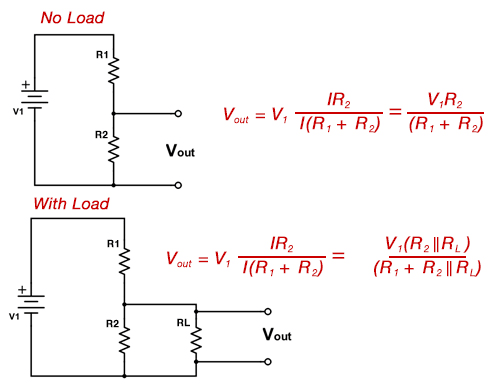# Voltage Divider Calculator

## About:

A voltage divider is a passive linear circuit that produces an output voltage (Vout) that is a fraction of its input voltage (V1). Voltage dividers are used to make signal level adjustments, for active device and amplifier bias, and for measuring voltages.Voltage Input (V1):
V
1st Resistance (R1):
Ω
2nd Resistance (R2):
Ω
Voltage Output (Vout):
V =

If Under Load

Load (RL):
Ω
Output Circuit Voltage:
V =
Power Supplied by the Power Supply:
W =
Power Delivered to the Load Resistor (RL):
W =
% of the Total Power the Load Receives:
% =